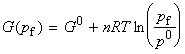Back to Table of Contents [TOP] Properties of the Gibbs free energy: [ TOP ] Properties of the Gibbs free energy We have defined the Gibbs free energy G and the enthalpy H as: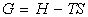and hence: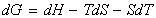i.e.:But for a system doing no non-expansion work, dU may be replaced by the fundumental equation of thermodynamics, i.e.:and hence we have: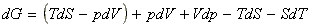which simplifies to:which since dG is an exact differential gives us: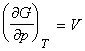and: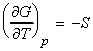These relationships show that G varies with temperature and pressure  [ TOP ] (1) The temperature dependence of the Gibbs free energy (The Gibbs-Helmholtz equation.) The temperature dependence of G is governed by the following relationship:This means that because S is positive, then G decreases when the temperature increases at constant pressure (G vs T plot, see fig. 1). Then sharpness of the decrease of G decreases with temperature at constant pressure is determined by the entropy of the system. This means that the sharpness of the decrease of the G vs T (i.e. the sensitivity of G with changes in T, = gradient of G vs T plot, see fig. 1) is greatest for a gas, then liquid, then solid. Let us now derive a relationship between the Gibbs energy and enthalpy (The Gibbs-Helmholz equantion). From the definition of G , we have:i.e.: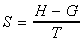i.e.: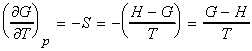which simplifies to the Gibbs-Helmholtz equation (see proof below):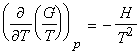The Gibbs-Helmholtz equation is most useful when applied to changes, including change of phase or chemical reactions at constant pressures. Then since DG = Gf - Gi, and since the Gibbs-Helmholtz equation applies to both Gf and Gi, we can write:Fig. 1: The variation of the Gibbs energy with the temperature is determined by the entropy.   Aside: Proof of the Gibbs-Helmholtz equation: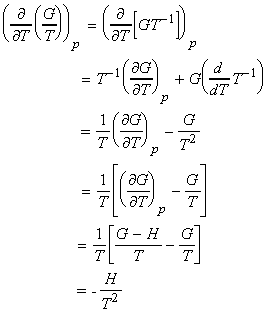[ TOP ] (2) The pressure dependence of the Gibbs free energy The pressure dependence of G is governed by the following relationship:This means that because V is positive, then G always increases when the pressure increases at constant temperature (G vs p plot, see fig. 2). Also, since V is the gradient of the G vs p plot, then the sensitivity of G to changes in p is greatest for gases and negligible for liquids and solids (see fig. 2).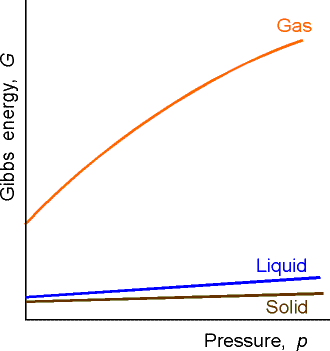Fig. 2: The variation of the Gibbs energy with the pressure    We can find the change in the Gibb's energy due to a change in pressure at constant temperature through: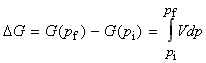For solids and liquids, we then treat V as constant, whilst for an ideal gas we use the pV=nRT relationship, and we get: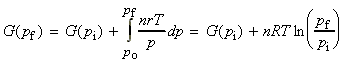or if we assume pi = p0 then we get: